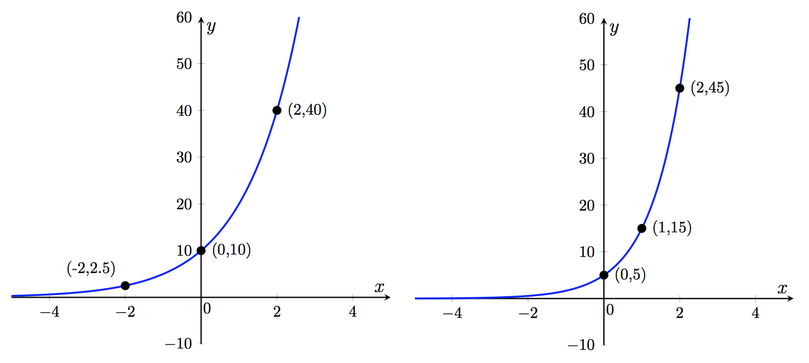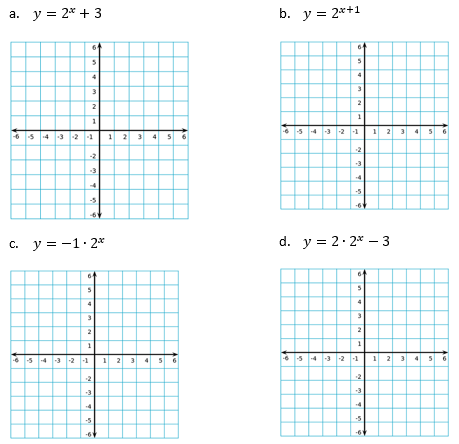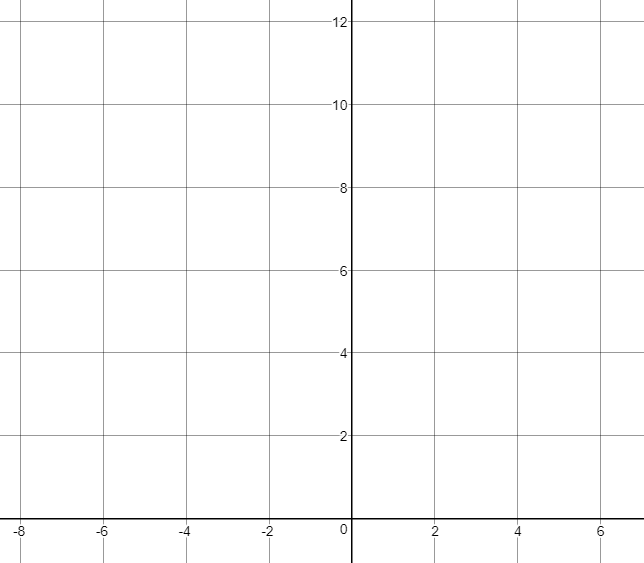# Exponents and Exponential Functions

## Objective

Graph exponential growth functions and write exponential growth functions from graphs.

## Common Core Standards

### Core Standards

?

• F.BF.B.3 — Identify the effect on the graph of replacing f(x) by f(x) + k, k f(x), f(kx), and f(x + k) for specific values of k (both positive and negative); find the value of k given the graphs. Experiment with cases and illustrate an explanation of the effects on the graph using technology. Include recognizing even and odd functions from their graphs and algebraic expressions for them.

• F.IF.C.7.E — Graph exponential and logarithmic functions, showing intercepts and end behavior, and trigonometric functions, showing period, midline, and amplitude.

?

• 8.F.B.4

## Criteria for Success

?

1. Analyze an exponential growth function in equation form to identify features in the graph of the function including the $y$-intercept, domain, range, rate of change, etc.
2. Analyze the graph of an exponential growth function to write an equation for the function.
3. Transform the graph of the parent function ${f(x)=a^x}$.

## Anchor Problems

?

### Problem 1

Consider the geometric sequence below.

${2,\space6,\space18,\space54,\space162, …}$

1. Write an explicit function, ${ f(x)}$, to represent the sequence. In this case, let the first term in the sequence correspond to ${ x=0}$.
2. What is the $y$-intercept of the graph of the function?
3. What coordinate point does each number in the sequence correspond to?
4. Sketch a graph of the exponential function given by the geometric sequence.

### Problem 2

Write a function in the form ${f(x)=a\cdot b^x}$ for each graph below.#### References

Illustrative Mathematics Identifying Exponential FunctionsPart B

Identifying Exponential Functions, accessed on May 17, 2018, 1:10 p.m., is licensed by Illustrative Mathematics under either the CC BY 4.0 or CC BY-NC-SA 4.0. For further information, contact Illustrative Mathematics.

Modified by The Match Foundation, Inc.

### Problem 3

The graph of the parent function ${{y=2^x}}$ is shown.For each function below, describe the transformation to the graph of the parent function ${{y=2^x}}$. Then sketch the graph.## Problem Set

?

The following resources include problems and activities aligned to the objective of the lesson that can be used to create your own problem set.

• Include examples where students match equations to graphs of exponential functions, and perform error analysis on mismatched equations and graphs

Sketch the graph of ${f(x)=2^{(x+3)}+4}$.